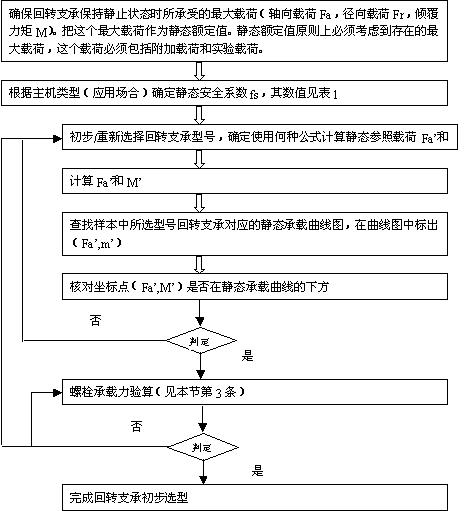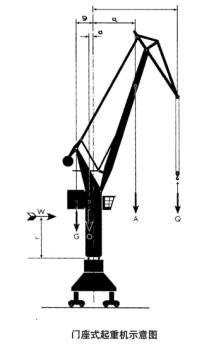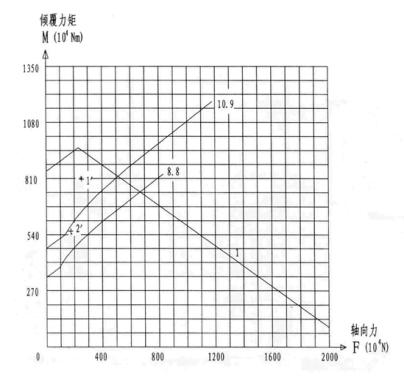• 6up官网_pokerstars安卓下载
• 地　址：河南省洛阳市宜阳轴承产业园区
• 销售部：0379-65216522　13703888536
• 技术部：0379-65216525　18736312885
• 供应部：0379-65216523　13383895999

# 回转支承型号选择计算方法

###### [ 发布日期：2014-12-26 点击：3821 来源:6up官网_pokerstars安卓下载 【打印此文】【关闭窗口】]

静态选型

1 ）选型计算流程图2 ）静态参照载荷 Fa' 和 M' 的计算方法：

·单排四点接触球式：

I、a=45° II、a=60°

Fa'=(1.225*Fa+2.676*Fr)*fs Fa'=(Fa+5.046*Fr)*fs

M'=1.225*M*fs M'=M*fs

·单排交叉滚柱式

Fa'=(Fa+2.05Fr)*fs

M'=M*fs

·双排异径球式

Fa'=Fa*fs

M'=M*fs

·三排滚柱式

Fa'=Fa*fs

M'=M*fs

·动态选型

·螺栓承载力验算：

·把回转支承所承受的载荷（没有乘静态安系数 fs ）作为选择螺栓的载荷。

·查对载荷是否在所需等螺栓限负荷曲线以下；

·若螺栓承载能力不够，可重新选择回转支承，或与我公司联系。

·选型计算距离 - 门坐式起重机（抓斗）·计八风力的工作载荷

2) 不计风力，考虑 25% 实验负荷的载荷

Q=260KN lmax=23m

A=75KN amax=11m

O=450KN o=0.75m

G=900KN g=3m

W=27KN r=6.5m

·八风力时的工作载荷：

Fa=Q+A+O+G=260+75+450+900=1685KN

M=Q*lmax+A*amax+W*r-O*o-G*g=260*23+75*11+27*6.5-450*0.75-900*3=3943KNm

2) 不计风力，考虑 25% 试验负载时的工作载荷

Fa=1.25Q+A+O+G=325+75+450+900=1750KN

M=1.25Q*lmax+A*amax-O*o-G*g=325*23+75*11-45*0.75-900*3=5566.3KNm

·不计风力时工作载荷

Fa=1685KN

M=Q*lmax+A*amax-O*o-G*g=260*23+75*11-450*0.75-900*3=3767.5KNm

Fa'=1750KN*1.45=2537.5KN

M'=5566.3KNm*1.45=8071.1KNm

Fa=1750KN

M=5566.3KNM?

l 线为静态承载能力曲线

8.8 、 10.9 为螺栓承载曲线

1'- 静态载荷点

2'- 螺栓载荷点

1' 点再滚道静态承载曲线 1 下方，因此满足要求

2' 点在 10.9 螺栓承载曲线下方，因此选择 0.9 螺栓可以满足要求。